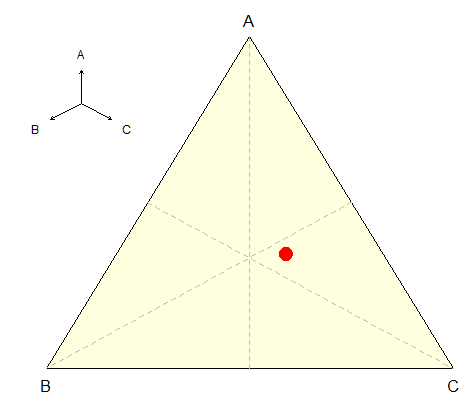Want to share your content on R-bloggers? click here if you have a blog, or here if you don't.

Consider here the case where, in some parametric inference problem, parameter  is a point in the Simplex,

For instance, consider some regression, on compositional data,

```> library(compositions)
>  data(DiagnosticProb)
>  Y=DiagnosticProb[,"type"]-1
>  X=DiagnosticProb[,c("A","B","C")]
>  model = glm(Y~ilr(X),family=binomial)
>  b = ilrInv(coef(model)[-1],orig=X)
>  as.numeric(b)
 0.3447106 0.2374977 0.4177917```

We can visualize that estimator on the simplex, using

```>  tripoint=function(s){
+    p=s/sum(s)
+    abc2xy(matrix(p,1,3))
+  }

>  lab=LETTERS[1:3]
>  xl=c(-.1,1.25)
>  yl=c(-.1,1.15)
>  library(trifield)
>  A=abc2xy(matrix(c(1,0,0),1,3))
>  B=abc2xy(matrix(c(0,1,0),1,3))
>  C=abc2xy(matrix(c(0,0,1),1,3))
>  plot(0:1,0:1,col="white",
+  xlim=xl,ylim=yl,xlab="",ylab="",axes=FALSE)
>  polygon(rbind(A,B,C),col="light yellow")
>  text(B,-.05,lab)
>  text(A,1.05,lab)
>  text(C,-.05,lab)
>  segments((A+C)/2,(A+C)/2,B,B,col="grey",lty=2)
>  segments((A+B)/2,(A+B)/2,C,C,col="grey",lty=2)
>  segments((B+C)/2,(B+C)/2,A,A,col="grey",lty=2)
>  points(tripoint(b),pch=19,cex=2,col="red")```If we want to compute a ‘confidence region’, we can either use Bayesian models (with a Dirichlet distribution as prior distribution), or use bootstrap. We will use here the second idea

```>  MB=matrix(NA,1e4,2)
>  for(sim in 1:1e4){
+    idx=sample(1:nrow(DiagnosticProb),
+    size=nrow(DiagnosticProb),replace=TRUE)
+  Y=DiagnosticProb[idx,"type"]-1
+  X=DiagnosticProb[idx,c("A","B","C")]
+  model = glm(Y~ilr(X),family=binomial)
+  MB[sim,]=tripoint(as.numeric(
+    ilrInv(coef(model)[-1],orig=X)))}```

To get some ‘confidence region’, we can then use the bagplot, to get either a region where 50% of the boostraped estimators are, or 95%,

```>  library(aplpack)
> P1=bagplot(MB[,1],MB[,2], factor =1.96, cex=.9,
+ dkmethod=2,show.baghull=TRUE)
> P2=bagplot(MB[,1],MB[,2], factor =0.67, cex=.9,
+ dkmethod=2,show.baghull=TRUE) ```

Then we can easily plot those two regions,

```>  plot(0:1,0:1,col="white")
>  polygon(rbind(A,B,C),col="light yellow")
>  text(B,-.05,lab)
>  text(A,1.05,lab)
>  text(C,-.05,lab)
>  polygon(P1\$hull.loop,col="yellow",border=NA)
>  polygon(P2\$hull.loop,col="orange",border=NA)
>  segments((A+C)/2,(A+C)/2,B,B,col="grey",lty=2)
>  segments((A+B)/2,(A+B)/2,C,C,col="grey",lty=2)
>  segments((B+C)/2,(B+C)/2,A,A,col="grey",lty=2)
>  points(tripoint(b),pch=19,cex=2,col="red")```